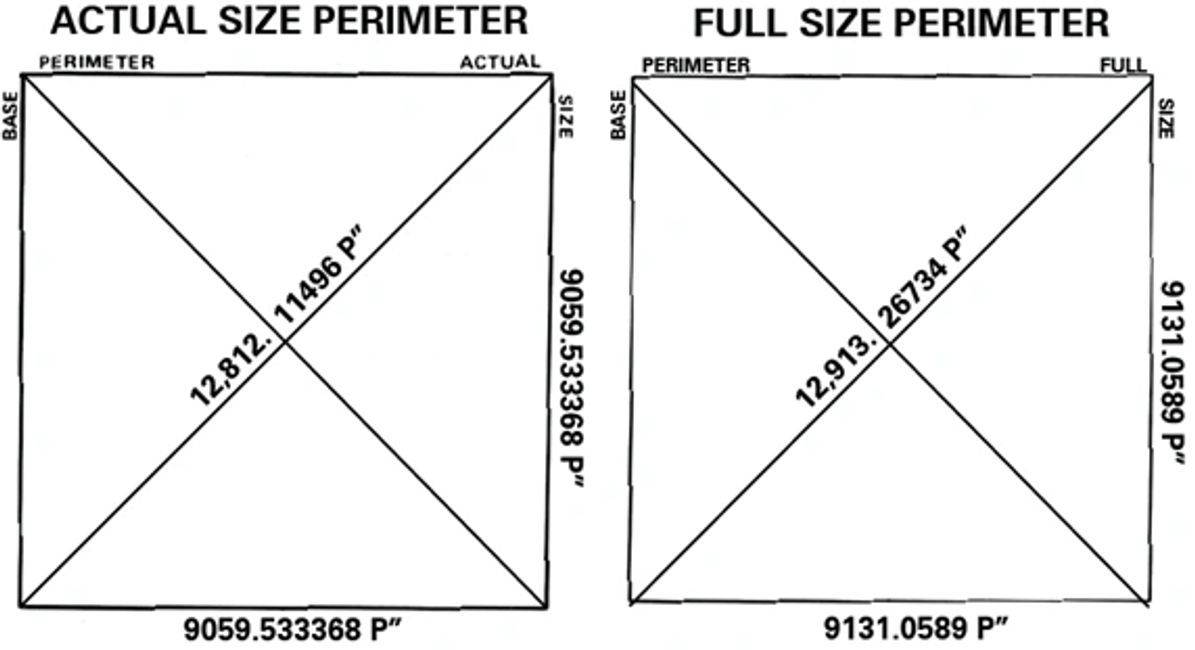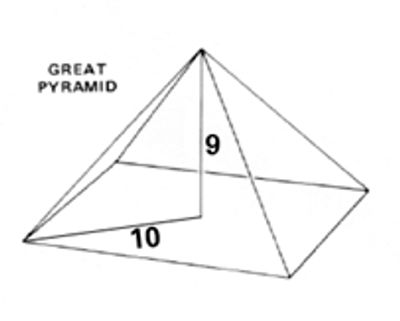# Scientific

The Great Pyramid has been said to express a number of scientific statements, although, in the author’s opinion not all can be proven. In the first volume it was shown that the Great Pyramid’s measurements were put forth in polar inches, polar cubits, and royal cubits. In that the Pyramid was designed and built with polar units of measure, it then makes reference to Earth’s polar diameter, which distance is demonstrated in its base. This fact necessitates that the designer knew the size of the planet and the polar flattening factor at the time of the Pyramid’s inception. The line of Earth’s axis, when extended into the north heavens, inscribes what we know as the precession of the equinoxes―a cycle that is nearly 26,000 years in length. If one adds the lengths of both the northwest/southeast and north-east/southwest diagonals of the Pyramid’s base, from corner to opposite corner, the calculation result for the as built actual size structure is 25,624.22992 P” as is shown in the drawing, “Actual Size Perimeter.” This same calculation, when applied to the theoretical full size Pyramid, would be 25,826.53468 P” as shown in the drawing, “Full Size Perimeter.” The full size distance was said by Sir Flinders Petrie’s father, the elder William Petrie, to have been the precession rate of the equinoxes. If one adds the Pyramid’s actual and full size diagonal distances, and divides by two, the mean result is 25,725.38230 P”, or 25,725 years. The period of the precession, the fixed ecliptic as given in C. W. Allen’s “Astrophysical Quantities,” is 25,725 years,  and is the same number of years obtained from the mean of the actual and full size diagonals of the Great Pyramid’s base. Is this result intended?

# PagesIt was also demonstrated in the first volume that the solar year, the sidereal year, and the relationship ratio between the two were encoded in the Great Pyramid’s base circuit. The fact that these values are there found would require the designer to have known that the orbital year progressively lengthens at the rate of 0.00000011 days per century, while the solar year shortens at 0.0000616 days per century. So the time and length of the orbital year would have been known as well.

In about 1880 A.D., the elder William Petrie further proposed that the Great Pyramid’s geometry expressed the distance from Earth to the sun of 91,800,000 miles. At the time, the scientific community thought this distance to be 95,233,000 miles and to represent one (1) AU, or the mean distance between Earth and the sun. In 1889 A.D. two groups of scientists presented their estimations for this distance, one being 90 to 91 million, and the other 92 to 93 million miles.  In the present day this one (1) AU distance is thought to be 92,955,807 miles,  and so W. Petrie’s Pyramid equivalent falls short by over a million miles. His Earth to sun Pyramid distance was derived by taking the height of the structure versus one-half of its diagonal base distance, which he said expressed a ratio of nine (9), the height of the structure, to ten (10), one-half the base diagonal, as referenced in the drawing, “9/10.” The theoretical nine (9) to ten (10) ratio distances, for the full size Pyramid, are respectively 5,813.012640 P” and 6,456.633670 P”. When the apex height distance is divided by the one-half base diagonal distance the result is not precisely 0.900000000, it is rather 0.9003163161. This number, 0.9003163161, is then the power that we put ten to. Ten to the ninth power, 10^9, equals 1,000,000,000, and this number multiplied by the apex height of the full size Pyramid, converted to British inches, to feet, and then to miles, equals 91,843,332 miles. However, if one takes ten (10) to the 9.003163161 power (109.003163161), the result is 1,007,310,036. One need take the height of the full size Pyramid to its apex in polar inches multiplied by the conversion factor of 1.001063285 to obtain British inches. The resultant product is then multiplied by 1,007,310,036, and divided by twelve (12) to find the number of inch-feet, which result is divided by 5,280 to determine the number of foot-miles. The full calculation is: [(5,813.012640 P” x 1.001063285 = 5,819.193529 B”) x (1,007,310,036 = 5.861732043 x 1012) ÷ (12 = 4.884776703 x 1011 feet) ÷ (5,280 = 92,514,710.28 miles)]. This result looks much like the distance that light travels from the surface of the sun to the surface of the earth at Earth’s mean orbit of 1 AU (astronomical unit), or 92,955,807 miles . Subtracting both the radius of the sun and that of Earth from 1 AU results in the distance that light travels from surface to surface. The sun’s shortest radius distance is 431,940 miles, and adding Earth’s radius of 3,963 miles sums to 435,903 miles. Subtracting these 435,903 miles from 1 AU, or 92,955,807 miles, results in 92,519,904 miles, and this distance minus the Pyramid’s calculation distance of 92,514,710 miles results in a 5,194 mile difference; that is, merely a 4/1000th or 0.004% difference—nothing at all. In fact, depending on the figure used for the sun’s diameter, 865,893 miles being the higher figure, or 863,880 miles the lower―both derived from 695,950 kilometers plus or minus 810 kilometers, results in answers differing by 1,006.62 miles for the radius. The distance from Earth to the sun similarly differs, with regard to our planet’s elliptical orbit around the sun, the range of which is between 91,403,259 and 94,512,259 miles for a variation band of 3,109,000 miles. The longest distance for the sun’s radius, when applied to the foregoing calculation, would change the light distance to 92,518,897 miles for a difference of only 4,187 miles from the Pyramid’s full size distance calculation. Earth’s orbital year is lengthening as we move forward in time, which means that the distance from Earth to the sun is expanding. At some time, not so long ago, the sun was 5,200 to 4,200 miles closer to Earth than it is today―just possibly the distance encoded in the Pyramid.

In the author’s mind the only scientific information the Pyramid need show is that required for an understanding of the structure as a chronograph. Those necessary concepts include knowledge that within the Pyramid there is a 286.1” distance expressed, and that the actual size Pyramid is short by 286.1 P” from the theoretical full size base perimeter, which information is required to understand that the base circuit encodes both the sidereal and solar year lengths. It is also required to have knowledge of the polar inch, the scored line date, the passage angle, intended distances, mathematical formulas, but little more. It is with these understandings that a chronograph of dates is manifest, and thereon are defined prophecies of God―the same prophecies recorded in the Old and New Testaments, thereby evidencing that the Bible and the Great Pyramid are works of one author, God. This is the most important knowledge expressed in the Great Pyramid, far more significant than the scientific knowledge that it holds, because God gave it to the world thru Enoch. Scientific knowledge is not necessarily evidence of God, but fulfilled prophecy is.  The world has been told a lie―an untruth; one that denies the existence of God, and insists that the Bible―the work of His hand, cannot be proven.